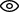### Create my accountLog in now or create an account to benefit from a fully personalized Unimy Masters experience

# Comprehensive GRE OverviewThe GRE is comprised of three core assessment areas:

• Analytical Writing
• Verbal Reasoning
• Quantitative Reasoning

These areas are designed to measure your aptitude for critical reasoning and quantitative analysis, and to assess your ability to write coherent, well-supported arguments based on available evidence and instructions.

The core assessment areas are spread out across the exam in five scored sections: one analytical reasoning section, two verbal reasoning sections, and two quantitative reasoning sections. You will have 30 minutes to complete each Analytical Writing prompt and Verbal Reasoning section, and 35 minutes for each Quantitative Reasoning section. The exam can take anywhere from three-and-a- half to four hours depending on the version of the exam administered.

## Analytical Writing

Your exam will consist of one scored Analytical Writing section that includes two prompts:

Analyze an Argument prompt

The Analyze an Argument prompt will present you with an argument and ask you to evaluate its merits and logical coherence. Unlike the Analyze an Issue prompt, you will not choose a side for this prompt. Instead, you will write a critical assessment of the argument presented. The Analytical Writing section will always appear first on the exam. You will have 30 minutes to complete each prompt. The prompts are separately timed, and you can only work on one prompt at a time. The section is scored on a scale of 0-6, in half-point increments. A 6 is the highest possible score.

Analyze an Issue prompt

The Analyze an Issue prompt will present you with specific instructions on how to analyze a given topic. The topic lends itself to multiple perspectives, and there is no correct answer. What is important is that you construct a well-reasoned, cohesive argument that both supports your stance on the issue and closely follows the instructions given in the prompt.

## Verbal Reasoning

In the two Verbal Reasoning sections, you will be asked to read and synthesize information presented in various forms from short sentences to multi-paragraph passages. This assessment area is designed to test your ability to comprehend and evaluate written material. The Verbal Reasoning sections also measure your understanding of sentence structure, punctuation, and proper use of vocabulary.

Your exam will consist of two scored Verbal Reasoning sections that include the following question types:

Reading Comprehension questions require you to read given passages and select the answer that best completes the question task. The passages’ content can come from a wide-range of subject matters, and there is often more than one question that corresponds to each passage.

Text Completion

Text Completion questions require you to identify the appropriate term (or terms) that best completes a given sentence. Text Completions can have anywhere from one to three terms that need to be identified. A strong vocabulary and the ability to understand context clues are both essential in this section.

Sentence Equivalence

Sentence Equivalence questions require you to identify two terms for a single blank in a sentence that will create two similar sentences that express the same main idea. Similar to the Text Completion questions, this section requires a strong vocabulary and command of context clues.

## Quantitative Reasoning

In this section, you will be asked to solve mathematical problems drawn from the subject areas of arithmetic, geometry, algebra, and data analysis. This section tests your ability to solve quantitative problems, understand real-world applications of mathematical principles, and interpret statistical data from charts and graphs.

Check out: How to Choose GRE and GMAT Test Dates

Your exam will consist of two scored Quantitative Reasoning sections that include the following question types:

Quantitative Comparisons

Quantitative Comparison questions require you to analyze the relationship between two given quantities and select the answer that best describes the relationship. These questions focus more on understanding mathematical relationships and less on actual mathematical calculations.

Mathematical Problem-Solving

Mathematical Problem-Solving questions require you to use various mathematical formulas and processes to work out the correct answer to the given problems. These are multiple choice questions that can have either one or multiple correct answers. These questions may also require you to input your own answer without providing you with any choice of answer.

Data Interpretation

Data interpretation questions require you to interpret data from charts and graphs in order to work out the correct answer. These questions are a sub-set of the Mathematical Problem-Solving questions and occur as part of a set where you will use one chart or graph to answer multiple questions.

Hopefully, this detailed overview will get you started on your preparation for the GRE exam.

Good luck!